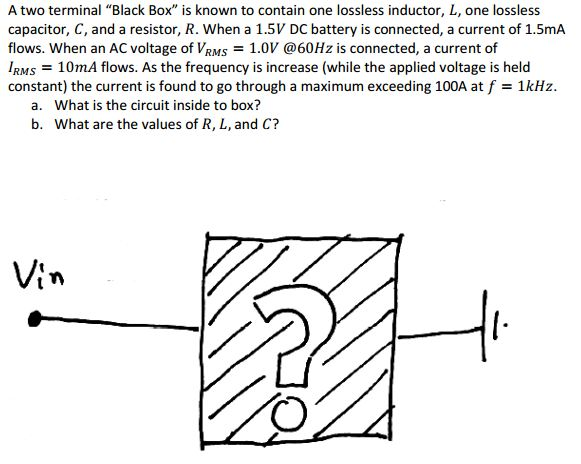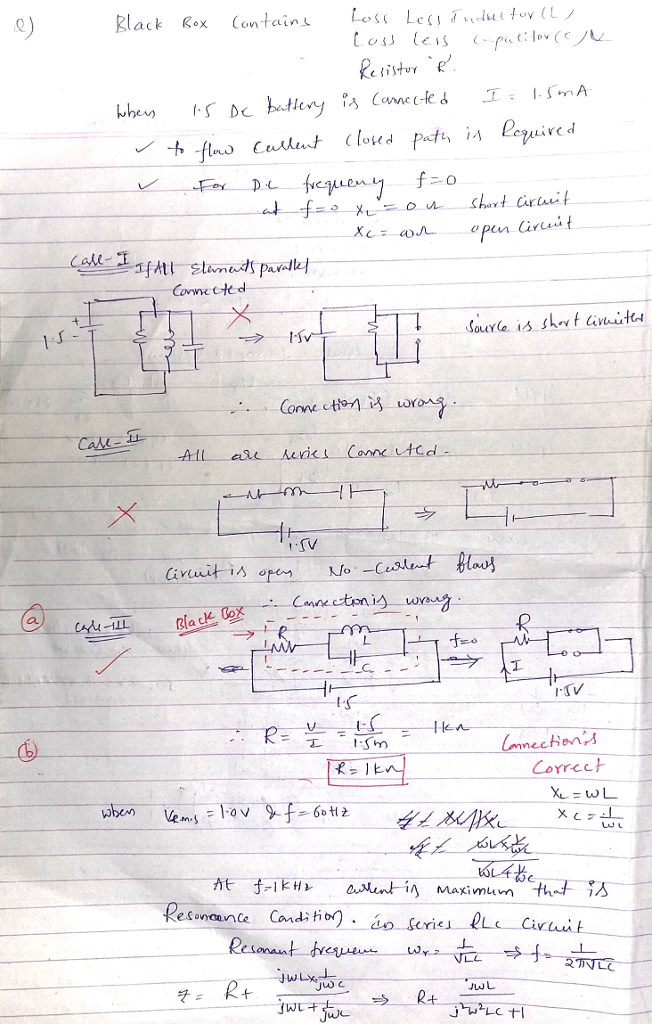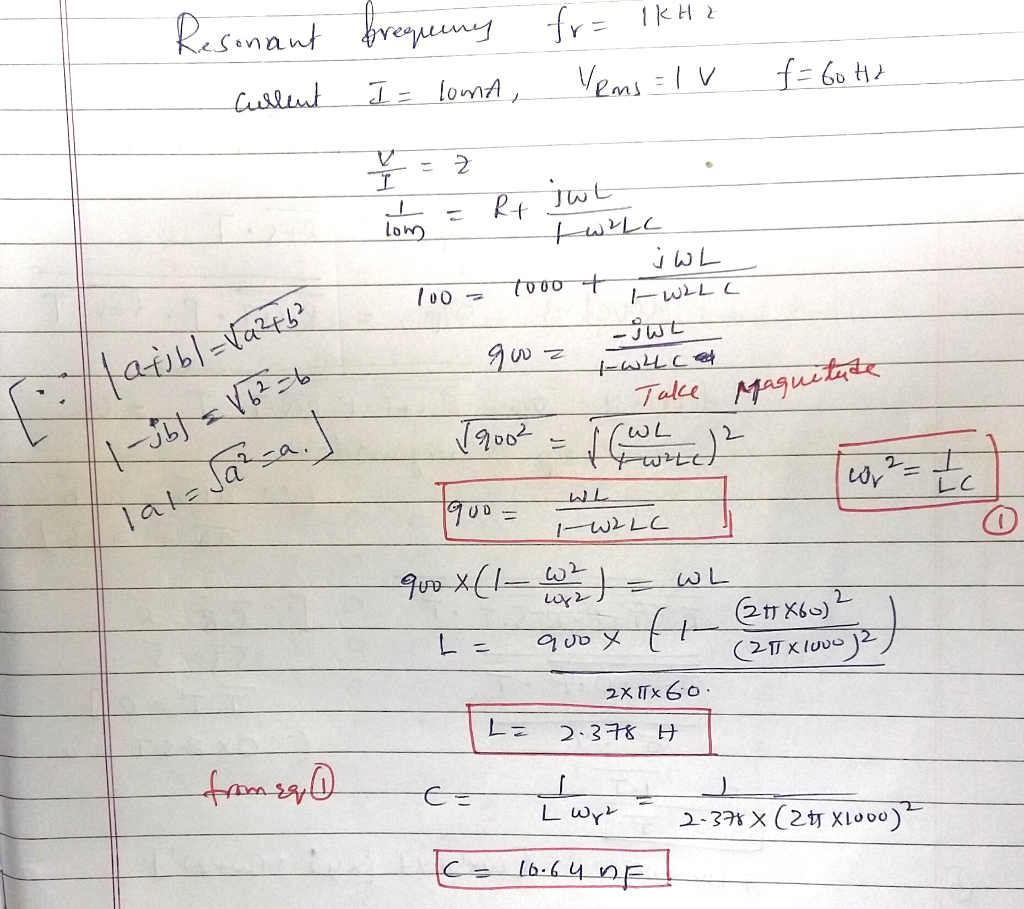# Homework Solution: A two terminal "Black Box" is known to contain one lossless inductor, L, one lossless capacitor, C, and…A two terminal "Black Box" is known to contain one lossless inductor, L, one lossless capacitor, C, and a resistor, R. When a 1.5V DC battery is connected, a current of 1.5mA flows. When an AC voltage of V_RMS = 1.0V @60Hz is connected, a current of I_RMS = 10mA flows. As the frequency is increase (while the applied voltage is held constant) the current is found to go through a maximum exceeding 100A at f = 1 kHz. a. What is the circuit inside to box? b. What are the values of R, L, and C?